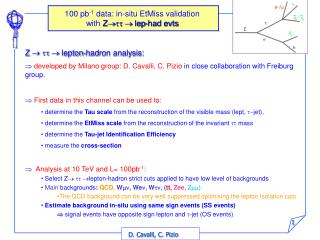DownloadDownload PresentationZ    lepton-hadron analysis:

# Z    lepton-hadron analysis:

Télécharger la présentation## Z    lepton-hadron analysis:

- - - - - - - - - - - - - - - - - - - - - - - - - - - E N D - - - - - - - - - - - - - - - - - - - - - - - - - - -
##### Presentation Transcript

1. 100 pb-1 data: in-situ EtMiss validation with Ztt lep-had evts • Z    lepton-hadron analysis: • developed by Milano group: D. Cavalli, C. Pizio in close collaboration with Freiburg group.  First data in this channel can be used to: • determine the Tau scale from the reconstruction of the visible mass (lept, t-jet), • determine the EtMiss scale from the reconstruction of the invariant tt mass • determine the Tau-jet Identification Efficiency • measure the cross-section •  Analysis at 10 TeV and L= 100pb-1: • Select Z  lepton-hadron strict cuts applied to have low level of backgrounds • Main backgrounds: QCD, Wmn, Wen, Wtn, (tt, Zee, Zmm) • The QCD background can be very well suppressed optimising the lepton Isolation cuts • Estimate background in-situ using same sign events (SS events) • signal events have opposite sign lepton and t-jet (OS events)

2. Analysis Method Two separated analysis  use the invariant mass to tune the EtMiss scale and the visible mass to tune the tau scale.  This analysis is now implemented in the Z/W Benchmark package 5.Separate OS evts from SS events Signal: only OS evts, Backgds: OS and SS with similar probabilitybackground contribution can be estimated in-situ using SS events 6. Subtract SS from OS evts A correction is needed for W background where OS/SS≈1.5  correction factor determined in situ (cfr. ATL-PHYS-INT-2009-005) • Select max pT lepton in the event pT>10 GeV(15) Use single ele/m trigger • Basic cut flow: - ETMiss>20 GeV -mandatory for MET scale determination -helpful against QCD and Zll - Lept – ETMiss Transverse Mass (mT) < 30(50) Gev - SET < 400GeV 3. Invariant/Visible mass reconstruction: use e/m candidate and t-jet candidates 7.  ETMiss scale determination from reconstruted invariant mass  t scale determination from reconstructed visible mass 4.Second cut flow: - 1. <Dj (Lept –t-jet) < 3.1 (2.8) - Invariant Masstt >0

3. Z tt:tune Missing ET Scale from invariant m OS all OS Signal SS all 1 2 OS-SS • tt invariant mass distributions: • 1) OS (signal + background), SS (signal + background), NO QCD, OS Signal • 2) OS – SS • In 100pb-1  200 Signal evts S/B=22 • S/B ~ 5 taking into account QCD background (still preliminar…) • QCD can be well suppressed optimizing the lepton isolation cut

4. Test of Ryan's optimisation for muons QCD background using very large statistics of AtlfastII events Isolation cut for muons: EtCone30 <= 2 && NuCone40 < 1 only muon channel

5. Z tt:tune Missing ET Scale from invariant m • Determination of the EtMiss scale with invariant m: • in 100 pb-1invariant m mass reconstructed with an error of less then 1 GeV (0.8 GeV) • taking into account only the statistical error the EtMiss scale could be determined with a precision of 3 % • taking into account also systematic effects (subtraction of the SS events and the stability of the fit..) EtMiss scale could be determined with a precision of 8 % preliminar +3 +1 -1 -3 Inv mass vs ETMiss Scale OS Signal evts OS-SS Signal+Backgd evts (the one that we will have in real data !) NO QCD included yet

6. BACK-UP SLIDES

7. Invariant tt Mass Reconstruction Ex= (En1*u1)x + (En2*u2 )x Ey= (En1*u1)y + (En2*u2)y m = 2(Elept+ E1)(Et-jet+ E2)(1 - cos) • Elept,Et-jet =energies of visible decay products = angle bet. the directions of visible decay products • E1, E2= energiesof the two neutrinos systems Assumptions: • m= 0 • collinearity The energies of the two neutrinos systems are obtained solving the system Containing the two EtMiss projections: • Determinant has to not be zero (sinDf≠0)  not back-to-bact lepton & t-jet • E1, E2have to be > 0 This system cannot be always solved The invariant mass cannot be always calculated

8. EtMiss Performance: Mass reconstruction ETmiss |sin ()| tt invariant mass reconstruction Generated mZ p.l. reconstructed mtt The reconstructed mtt is dominated by EtMiss measurement: EtMiss linearity and resolution crucial for tt mass reconstruction Collinearity hypothesis All reco quantities ETMiss calc from particles in |h|<5) reconstructed ETMiss Effect of detector acceptance Effect of EtMiss reconstruction s(m) 

9. Subtraction of backgrounds in-situ using SS events W+jets • Main backgrounds are QCD, W+jets: • QCD: same probability for OS and SS • W+jets: OS/SS=1.5 in pp two production channels (qq’->SS/OS, qg->OS) • Procedure to evaluate number of W OS evts: Do not apply the mTlep-METcut • From mTlep-MET distribution at the end of cuts : - Evaluate from data the number of W SS (NSSCONT)and OS events in the W control region (RSOS = OS/SS):  for 50<mTlep-MET (GeV)<100 only W events are collected (other evts/W evts= 2.5%) Get from MC the fraction of W evts with mTlep-MET < 30 GeV in signal region respect to control (RCONT= from MC ) compare different MC… 3) Evaluate the number of W events OS expected in Signal region: NOSSignal region=RCONT*RSOS* NSSCONT Signal region W control region

10. What can we do with 50pb-1 ? 50pb-1 100pb-1